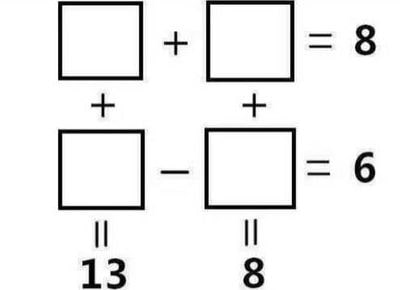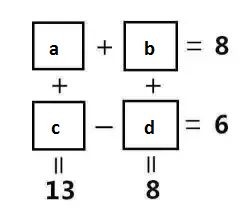Can you fill in the numbers in the boxes and solve the puzzle?a = 3.5
b = 4.5
c = 9.5
d = 3.5

To solve the puzzle, you will need to come up with 4 equations:
a + b = 8 … (1)
c – d = 6 … (2)
a + c = 13 … (3)
b + d = 8 … (4)

Subtracting (1) from (3) gives:
c – b = 5 … (5)

b + c = 14 … (6)

2c = 19
c = 9.5

Substituting c = 9.5 in (2) gives:
9.5 – d = 6
d = 3.5

Substituting d = 3.5 in (4), gives:
b + 3.5 = 8
b = 4.5

Substituting b = 4.5 in (1), gives:
a + 4.5 = 8
a = 3.5

« Previous Riddle
Next Riddle »